# Polynomial Construction from Sequences IV

Don't feel obligated to understand any of the content below. The complexity of this post is very high and I put this here to show how much more difficult it can be to solve a problem if you don't tackle it from the correct perspective. In the last post, some insight was found on how to find a diagonal row of Pascal's triangle numbers, and a Haskell program was written to find polynomials out of number sequences to make life a lot easier. Now it's time to examine the results of the 2nd post of this series.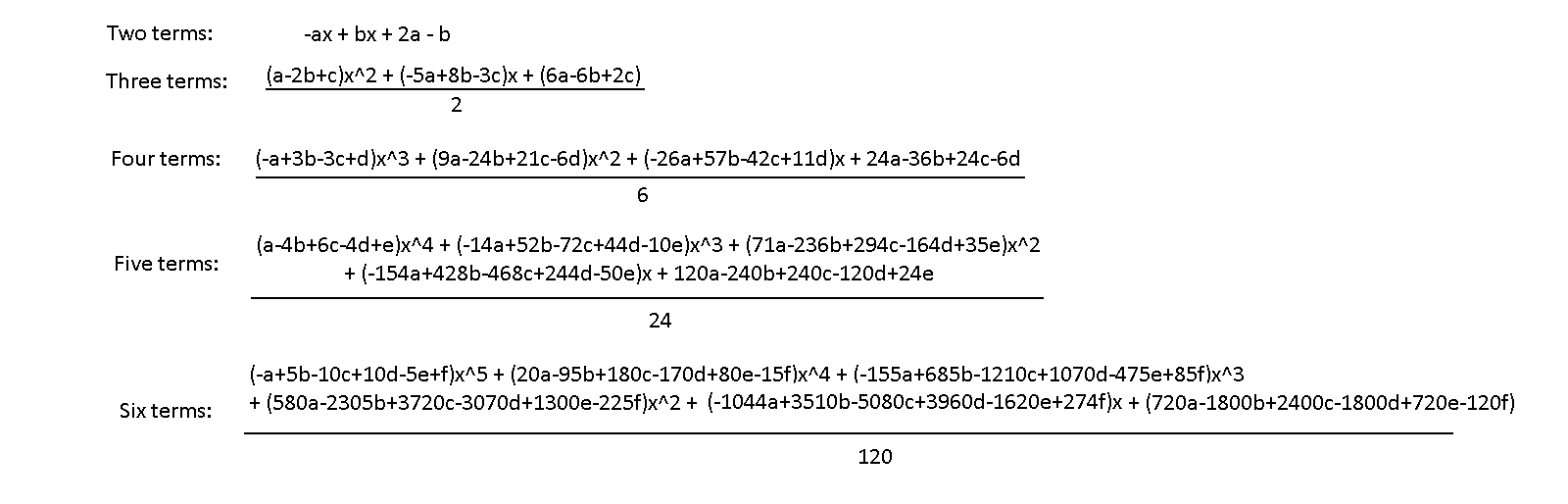One thing to note is that every term except for the last term in the series has coefficients that sum to 0. For example, if you were to set all the letters equal to the same value in the 5 term expression, then the only part that wouldn't be zero is the x^0 term. The x^0 terms in each of these expressions equate to some value of a factorial function.

If you had any linear sequence of numbers, ex. 1,2,3,4, etc., then plug them into each variable a,b,c,d, etc., of the expressions, all but the last TWO terms will sum to 0. This makes sense if you think about it, since each of these terms are designed to "detect" a specific kind of difference pattern in a number sequence. The higher-order terms like x^2 wouldn't detect a pure linear sequence, but they could detect a quadratic or cubic sequence.

Another thing that pops out is the formation of Pascal's triangle on the first term of each polynomial. The difference is that every other term is negative. The other terms in any of the other series exhibit a pattern similar to that of a row of pascal's triangle, where the coefficients increase near the middle, and then they decrease. The nature of the lower-power terms in the expressions is dependent on the previous terms, since to generate those terms we used the results of the higher-power terms. So, we are likely not going to get any nice formula to generate an entire expression at once: it will be a recursive definition.

Let's start with finding the first terms of a sequence, which is also the first step in creating these expressions. We know that the coefficients form values of rows of Pascal's triangle. This means we know a quick way to generate these values already: the combination function. Below is an example of the notation and usage. Here we generate the third row of Pascal's triangle.There's just a couple things missing. First of all, each term flips its sign, such as -a + 3b -3c + d. Not only that, but each sequences also flips all of its terms: the four term expression is a - 4b + 6c - 4d + e. So, the variable's sign depends on both its position in a term, and on which expression it resides in.

Time to get serious with the formalities here. So, instead of labeling the variables inside each term as letters of the alphabet, I'm going to name them C0, C1, C2, etc…, and N will be what polynomial order we are dealing with, or what term-expression we are trying to generate. Also, all of these expressions have all their terms divided by some number, which grows factorially. Taking all of this into account, here's the formula to use: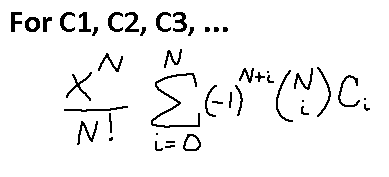Remember that this is only for the highest-order term. That's why X^N is there on the outside. We took into account the fraction of N!, as well. The (-1)^(N+i) part will take into account whether CN should be negative, and (N, i) is our combination function from earlier. Time to test this out for a 3-term sequence, which will be a 2nd order polynomial, hence why N = 2.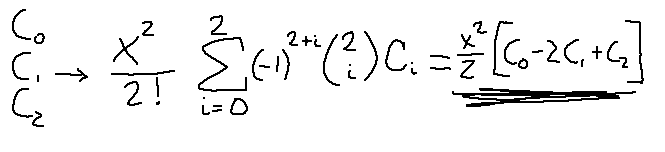Yep! The result matches what was expected. I equated that result to some y term so that I have an easier way of referencing the result later. Now, how to generate the rest of the terms? Well, in the second post of this series what we did next was take the original sequence and subtract it by this formula like so: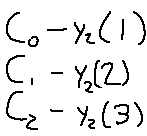Since the output of the above is yet another sequence, we can actually feed this back into the formula created earlier! We know that this sequence should generate a linear polynomial, so we just need to set N = 1 and feed only 2 of the terms. By doing this, we actually get the next correct term, (-5C1 + 8C2 - 3C3)(x/2).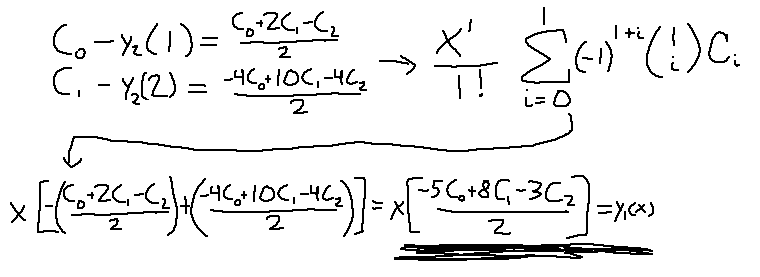Since the last term we know is going to be a constant, there's no need for plugging that one term into the formula, as we'll just get back out the same thing. So, all that's needed is to perform the subtractions and we have our whole expression.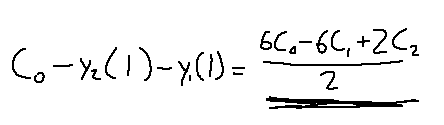What an even lengthier process than before, but at least we are getting closer to generalizing this entire thing.

We know how to find the highest-power term of a sequence, and we know how to subtract the original sequence by other sequences to output sequences with lower-powers. If we continuously repeat this process, we'll keep finding all the lower-power terms until we reach to the last one, the constant. Then, we have found the entire expression. It's time to bring this all together. First, we need a more concise way of representing the subtraction of y(x) terms from the original sequence…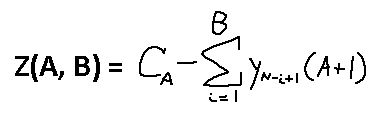Yeah, that'll work. When B = 0, you get back the CN value. When B = 1, 2, etc. you increase the number of terms to subtract from CN. Tuning A gives you a different CN to work with. Here's a concrete example of what all this would look like: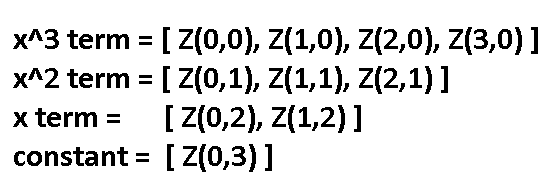Remember that in order to successfully find a sequence's Nth power term, we need N+1 sequence numbers in order to find it. This is why as the power terms get lower we require less and less of the original sequence.

Time to bring everything we have learned together. What kind of final form does this beast taken on? Well, technically there's four of them…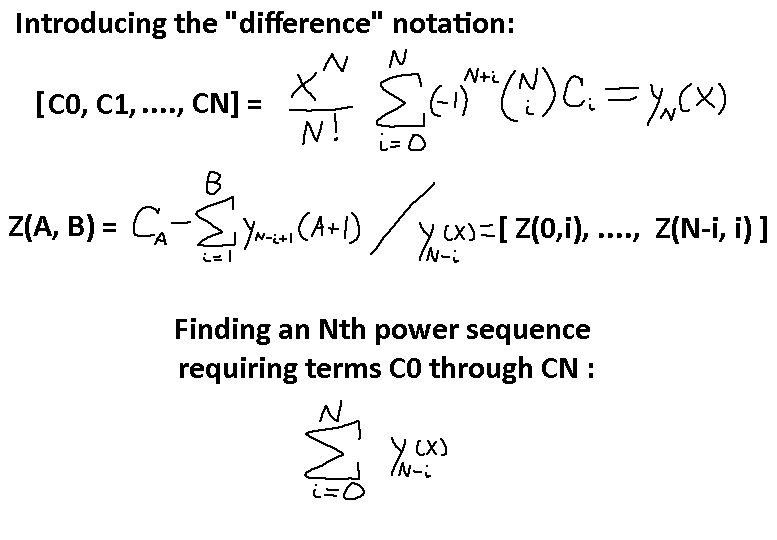These are the blueprints to generate any Nth power expression, where we can plug in any N + 1 numbers into the formula and get back a polynomial equation that passes through those points for x = 1, 2, 3, etc. Time for a sanity check! Using N = 2 because wow this is going to be a lot of work.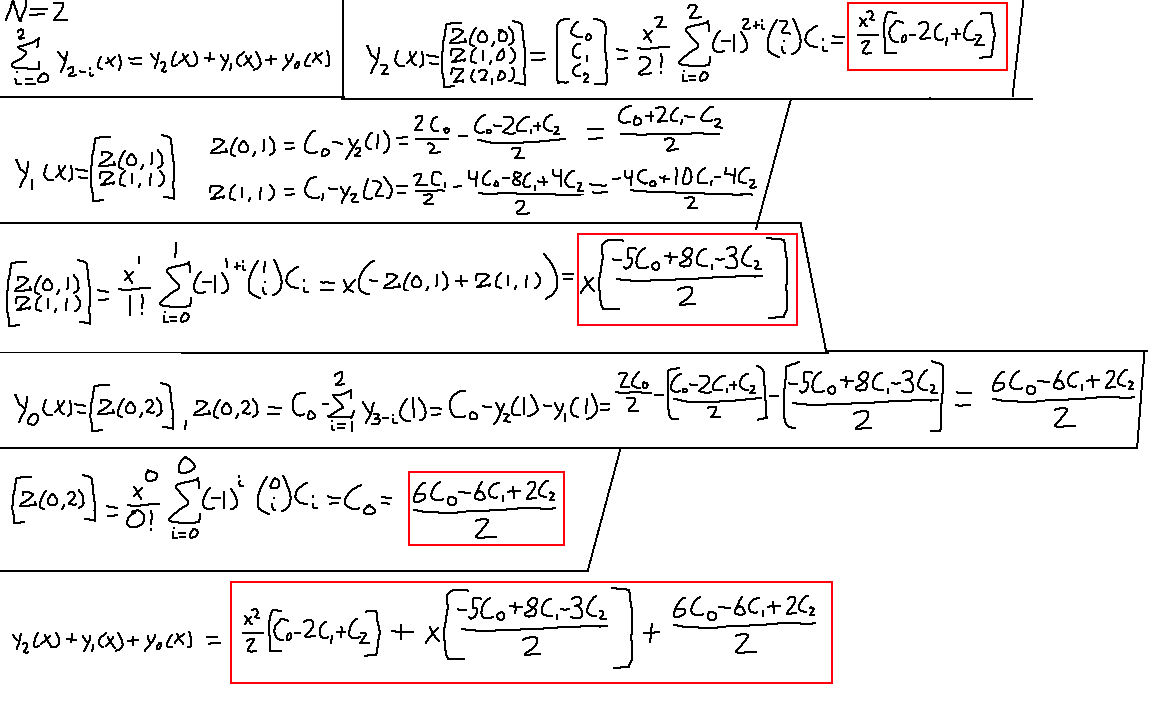Jeez… well, there it is. It matches up with the three-term form in the first image of this post.

On the next post, we do some restructuring of the terms and find a way to simplify this formula greatly.